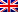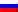# Linear programming

Contenus

## Linear programming

In Operational Research (OR) such as linear programming, modeling a problem consists in identifying:

• intrinsic variables (unknowns)
• the various constraints to which these variables are subject
• the targeted objective (optimization) called the Objective function.

In a linear programming (PL) problem the constraints and the objective are linear functions of the variables. We also speak of a linear program. They are noted as follows (pure canonical form):

where x is the vector of variables (x1,…, Xnot), vs and b are vectors of coefficients, TO a size coefficient matrix m * n, and T the transpose (column vector instead of row vector for example). Inequalities Ax ≤ b and x ≥ 0 (positivity of variables) are constraints. The defined domain of definition is a convex polytope. The linear program is said to be in standard form if it concerns equality constraints.

Positivity of variables. If in the context, we have a non-zero lower bound x ≥ k, just ask y = xk and y ≥ 0. If there is no lower bound, we can always set x = yz with y ≥ 0 and z ≥ 0.

Any standard problem can be written canonically and vice versa. The equality constraint breaks down into two inequalities constraints. An inequality constraint is written in the form of equality by adding a difference variable.

A solution x 'is said to be feasible if it satisfies all the constraints.

To sum up, linear programming is made up of four elements:

1. decision variables;
2. an objective function;
3. resource constraints;
4. "type" constraints.

To formulate a problem from a specification, follow the four previous points in order.

Example. Consider a factory that produces two types of keyboard earning £ 50 and £ 70 each. To manufacture a type 1 unit, it takes 40 min of labor and 20 min of machining. To manufacture a type 2 unit, it takes 30 minutes of labor and 30 minutes of machining. The workforce works 6 hours a day while the machine is available 8 hours a day. How many units of each type must be produced to maximize profits?

Linear program. This problem is modeled according to the following PL:

maximum 50x + 70y
SC 40x + 30y ≤ 360
20x + 30y ≤ 480
x, y≥ 0

## Possible solutions

Only four cases can arise:

1. If the domain of definition is null, the problem is not feasible;
2. otherwise: the optimum does not exist, the problem is unbounded;
3. or: the problem has a unique optimal solution coinciding with an extreme point (a vertex) of the domain of definition;
4. or: the problem has an infinity of solutions forming a face or an edge of the domain of definition.FRFRENRUES